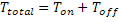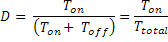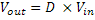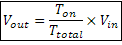Pulse width Modulation or PWM is one of the powerful techniques used in control systems today. It is used in wide range of application which includes: speed control, power control, measurement and communication. This tutorial will take you through basics of Pulse width modulation and its implementation on microcontrollers.

## Basic Principal of PWM

Pulse width modulation is basically a square wave with a varying high and low time. A basic PWM signal is shown in the figure below.

Pulse width modulation wave

There are various terms associated with PWM:

1. On-Time: Duration of time signal is high
2. Off-Time: Duration of time signal is low
3. Period: It is represented as the sum of on-time and off-time of PWM signal
4. Duty cycle: It is represented as percentage of time signal remains on during the period of the PWM signal

### Period

As shown in the the figure, Ton denotes the on-time and Toff denotes the off time of signal. Period is the sum of both on and off times and is calculated as shown in the equation below:### Duty Cycle

Duty cycle is calculated as on-time to the period of time. Using the period calculated above, duty cycle is calculated as:## PWM: Voltage Regulation

PWM signal when used at a different duty cycles gives a varying voltage at the output. This method is used in various areas of application like:

• Switching regulators
• LED dimmers
• Audio
• Analog signal generation
• and many more...

Voltage regulation is done by averaging the PWM signal. Output voltage is represented by the following equation:As you can see from the equation the output voltage can be directly varied by varying the Ton value.

If Ton is 0, Vout is also 0. if Ton is Ttotal then Vout is Vin or say maximum.

## Implementing PWM on 8051

The basic idea behind PWM implementation on 8051 is using timers and switching port pin high/low at defined intervals. As we have discussed in the introduction of PWM that by changing the Ton time, we can vary the width of square wave keeping same time period of the square wave.

We will be using 8051 Timer0 in Mode 0. Values for high and low level will be loaded in such a way that total delay remains same. If for high level we load a value X in TH0 then for low level TH0 will be loaded with 255-X so that total remains as 255.

### Assembly Code Example

#### Timer setup for PWM

```	PWMPIN EQU P1.0	; PWM output pin
PWM_FLAG EQU 0	; Flag to indicate high/low signal

PWM_SETUP:
MOV TMOD,#00H ; Timer0 in Mode 0
MOV R7, #160	; Set pulse width control
; The value loaded in R7 is value X as
; discussed above.
SETB EA ; Enable Interrupts
SETB ET0 ; Enable Timer 0 Interrupt
SETB TR0 ; Start Timer
RET
```

#### Interrupt Service Routine

As we are using Timer0 for generating PWM, timer interrupt service routine uses PWM_FLAG bit to selects the high and low section of PWM signal. When timer overflows, PWM_FLAG is checked. If flag is set timer is loaded with timer value for low time and if flag is cleared timer is loaded with high time value.

```TIMER_0_INTERRUPT:
JB PWM_FLAG, HIGH_DONE	; If PWM_FLAG flag is set then we just finished
; the high section of the
SETB PWM_FLAG		; Make PWM_FLAG=1 to indicate start of high section
SETB PWMPIN		; Make PWM output pin High
MOV TH0, R7		; Load high byte of timer with R7
; (pulse width control value)
CLR TF0			; Clear the Timer 0 interrupt flag
RETI			; Return from Interrupt to where
; the program came from
HIGH_DONE:
CLR PWM_FLAG		; Make PWM_FLAG=0 to indicate start of low section
CLR PWMPIN		; Make PWM output pin low
MOV A, #0FFH		; Move FFH (255) to A
CLR C			; Clear C (the carry bit) so it does
; not affect the subtraction
SUBB A, R7		; Subtract R7 from A. A = 255 - R7.
MOV TH0, A		; so the value loaded into TH0 + R7 = 255
CLR TF0			; Clear the Timer 0 interrupt flag
RETI			; Return from Interrupt to where
; the program came from
```

As everything is handled in ISR. so to stop PWM you can simply disable the timer.

```PWM_STOP:
CLR TR0			; Stop timer to stop PWM
RET
```

The width of PWM can be changed by changing the value of R7 register. In above example I am using 160, you can choose any value from 0 to 255. R7 = 0 will give you o/p 0V approx and R7 = 255 will give you 5V approx.

You can also make use of Timer1 if you want. And the output pin can be changed to whatever pin you want.

### C Code Example

As explained in assembly example, the same code can be implemented in C.

```/* Global variables and definition */
#define PWMPIN P1_0

unsigned char pwm_width;
bit pwm_flag = 0;

void pwm_setup()
{
TMOD = 0;
pwm_width = 160;
EA = 1;
ET0 = 1;
TR0 = 1;
}

/* Timer 0 Interrupt service routine */
void timer0() interrupt 1
{
if (!pwm_flag) {	/* Start of High level */
pwm_flag = 1;	/* Set flag */
PWMPIN = 1;		/* Set PWM o/p pin */
TH0 = pwm_width;	/* Load timer */
TF0 = 0;		/* Clear interrupt flag */
} else {			/* Start of Low level */
pwm_flag = 0;	/* Clear flag */
PWMPIN = 0;		/* Clear PWM o/p pin */
TH0 = 255 - pwm_width;	/* Load timer */
TF0 = 0;		/* Clear Interrupt flag */
}
}

void pwm_stop()
{
TR0 = 0;			/* Disable timer to disable PWM */
}
```

## AVR Timers as PWM

Most of AVR controllers have onchip PWM channel which makes PWM usage much simple and more accurate. AVR timers or counters can be used in PWM mode without disturbing the basic timer function. As in case of AT90S8515, Timer1 can be configured in PWM mode by setting PWM11 and PWM10 bits in TCCR1A register. Following modes are available for PWM:

PWM11 PWM10 Description
0 0 PWM operation of Timer/Counter1 is disabled
0 1 Timer/Counter1 in 8-bit PWM Mode
1 0 Timer/Counter1 in 9-bit PWM Mode
1 1 Timer/Counter1 in 10-bit PWM Mode

The pre-scalar source for Timer/Counter1 can be selected with the help of clock select bits in TCCR1B register (more information please check datasheet at page 37).

Width of pulse is loaded in the timer output compare registers OCR1A (OCR1AH & OCR1AL) and OCR1B (OCR1BH & OCR1BL). Timer/Counter1 acts as an up/down counter, counting up from \$0000 to TOP (see table below), where it turns and counts down again to zero before cycle is repeated. When the counter value matches the content of 10 least significant bits of OCR1A or OCR1B, the PD5 (OC1A)/OC1B pins are set or cleared according to the settings of COM1A1/COM1A0 or COM1B1/COM1B0 bits in Timer/Counter1 Control register (TCCR1A), see table below.

PWM Resolution Timer Top Value Frequency
8-bit PWM \$00FF (255) Ftck1/510
9-bit PWM \$01FF (511) Ftck1/1022
10-bit PWM \$03FF (1023) Ftck1/2046
COM1X1 COM1X0 Effect on OCX1
0 0 Not Connected
0 1 Not Connected
1 0 Cleared on compare match, up-counting. Set on compare match down-counting (non-inverted PWM)
1 1 Cleared on compare match, down-counting. Set on compare match up-counting (inverted PWM)
Note: X can be A or B

### AVR: Assembly Code Example

```;8-bit Non-Inverted PWM code example
.equ pulse_width = \$40
;Pulse width can be changed from 0 to TOP
PWM_START:
ldi temp, pulse_width	;Load pulse width
out OCR1AL, temp	;OCR1A = Pulse width
clr temp
out OCR1AH, temp

ldi temp, \$81		;8-bit PWM Mode
out TCCR1A, temp	;Non Inverted

in temp, DDRD		;Make PortD.5 as o/p
ori temp, (1<<5)
out DDRD, temp

ldi temp, \$1		;Start PWM
out TCCR1B, temp
;PWM will run in background automatically
```

### AVR: PWM Setup in C

```/* Global variables and definition */
#define PULSE_WIDTH 0x40

void pwm_start()
{
OCR1AL = PULSE_WIDTH;	/* Load Pulse width */
OCR1AH = 0;
DDRD |= (1<<5);		/* PortD.5 as o/p */
TCCR1A = 0x81;		/* 8-bit, Non-Inverted PWM */
TCCR1B = 1;		/* Start PWM */
}
```

Most of AVRs have same set of registers for PWM setup. Just go through the datasheet once and configure registers. If you still feel any problem working on things, we are here to help. But please make use of forum

## Help & Queries

If you have any queries, doubts or feedback on this tutorial please share in our discussion forum. If you want us to write tutorial for more devices please let us know in the forum.